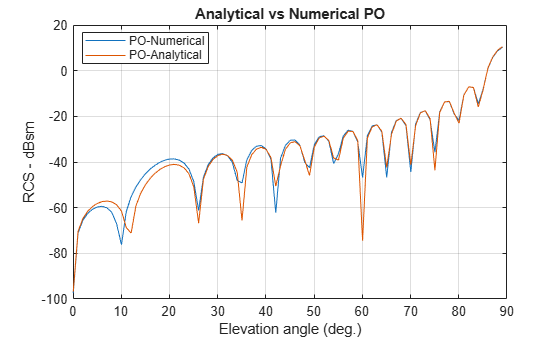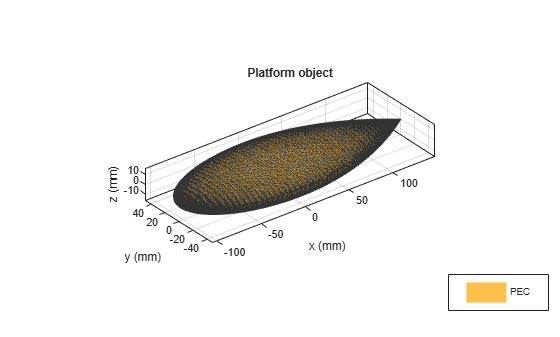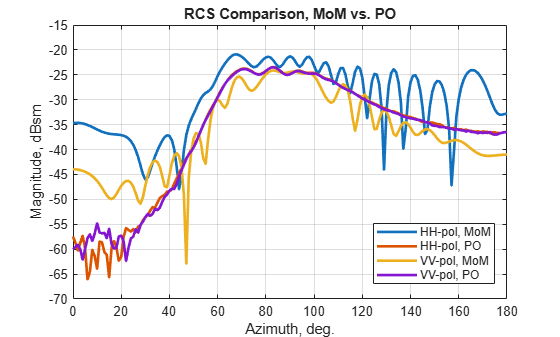Documentation

This example performs benchmarking of the Radar Cross Section computation on three structures: a square plate, a circular plate and the NASA almond. The benchmarking for the square and circular plate is done against the analytical physical optics based solution and in the case of the NASA Almond, the comparison is with the Method of Moments (MoM) solution.

### Square Plate RCS Parameters Setup

Define the physical dimensions for the square plate, the wavelength and frequency for analysis. The plate is defined using an STL file.

```lambda = 3.25e-2; f1 = physconst('lightspeed')/lambda; L = 10.16e-2; W = 10.16e-2; p = platform; p.FileName = 'square_plate.stl'; p.Units = 'm'; ```

### Analyze and Compare with Analytical result

The RCS computation is done in the elevation plane at azimuth = 0 deg. The electric-field polarization vector is set to HH. This implies a horizontal component on transmit and horizontal component on receive is used for the RCS calculation. The results for the RCS from the toolbox are compared with the analytical results provided in .

```az = 0; el = 0.05:1:90; sigma = rcs(p,f1,az,el,'Polarization','HH'); asigma1 = rectPlateRCS(L,W,f1,az,90-el); figure plot(el,sigma,el,asigma1) grid on xlabel('Elevation angle (deg.)') ylabel('RCS - dBsm') title('Analytical vs Numerical PO') legend('PO-Numerical','PO-Analytical','Location','best') ```### Circular Plate RCS Parameters Setup

Define the physical dimensions of the circular plate. The circular plate is described using an STL file. All dimensions are in meters.

```R = 10.16e-2; pc = platform; pc.FileName = 'circular_plate.stl'; pc.Units = 'm'; ```

### Analyze and Compare with analytical result

As before compare the results from the RCS function in the toolbox with the analytical expression provided in . The RCS calculation is done in the elevation plane between 0 and 90 deg.

```az = 0; el = 0.05:1:90; sigmaV = rcs(pc,f1,az,el,'Polarization','HH'); asigma1 = circPlateRCS(R,f1,90-el); figure plot(el,sigmaV,el,asigma1) grid on xlabel('Elevation angle (deg.)') ylabel('RCS - dBsm') title('Analytical vs Numerical PO') legend('PO-Numerical','PO-Analytical','Location','best') ```### NASA Almond Setup

The third structure is the NASA almond shape described in . This is a classic shape for benchmarking the performance of high-frequency electromagnetic solvers. The mathematical expressions in  have been used to create the STL file that describes the almond shape.

```p = platform; p.FileName = 'NASA-Almond.stl'; p.Units = 'm'; figure show(p) ```### Analysis parameters

Since the physical optics solver is only applicable at large ka values, we compare the results produced by the Antenna Toolbox with those published in . The results produced by the toolbox will be from both solvers, the physical optics (PO) and the Method-of-Moments(MoM). The wavelength at 7 GHz is approximately 4.3 cm. We refine the mesh to be slightly finer than this using thecriterion.

```f2 = 7e9; m = mesh(p,'MaxEdgeLength',.0035) az = 0:1:180; el =0; ```
```m = struct with fields: NumTriangles: 7878 NumTetrahedra: 0 NumBasis: [] MaxEdgeLength: 0.0035 MeshMode: 'manual' ```

### RCS Calculation with HH-Polarization

Calculate the RCS for the HH-polarization condition in which the transmitted and received field is horizontally polarized.

```sigmahh_po = rcs(p,f2,az,el,'Solver','PO',... 'EnableGPU', false,... 'Polarization','HH'); sigmahh_mom = rcs(p,f2,az,el,'Solver','MoM', ... 'Polarization','HH'); ```

### RCS Calculation with VV-Polarization

Calculate the RCS for the VV-polarization condition in which the transmitted and received field is vertically polarized.

```sigmavv_po = rcs(p,f2,az,el,'Solver','PO',... 'EnableGPU', false,... 'Polarization','VV'); sigmavv_mom = rcs(p,f2,az,el,'Solver','MoM', ... 'Polarization','VV'); ```

### Plot the results

Overlay the plots from both solvers for both polarizations to compare. Notice that the full-wave MoM solver extracts all the phenomenon that contribute to the scattered field. In contrast the PO solver being a first-order approximation is able to predict the level of RCS but averages out the variations at the different angles. This is expected since the PO solver assumes that the surface current density outside the illuminated region, i.e. the shadow region is zero, thus not contributing to the scattered field.

```figure plot(az,sigmahh_mom,az,sigmahh_po,az,sigmavv_mom,az,sigmavv_po,'LineWidth',2) ax = gca; ax.YLim = [-70,-15]; title('RCS Comparison, MoM vs. PO') xlabel('Azimuth, deg.') ylabel('Magnitude, dBsm') grid on legend('HH-pol, MoM','HH-pol, PO', 'VV-pol, MoM','VV-pol, PO','Location','best') ```### Summary

The RCS benchmarking results compare favorably with published results using analytical techniques as well as other numerical solvers.

### References

 Radar System Analysis and Design Using MATLAB, Bassem R. Mahafza, Chapman&Hall/CRC,2000.

A. C. Woo, H. T. G. Wang, M. J. Schuh and M. L. Sanders, "EM programmer's notebook-Benchmark radar targets for the validation of computational electromagnetics programs," in IEEE Antennas and Propagation Magazine, vol. 35, no. 1, pp. 84-89, Feb. 1993.